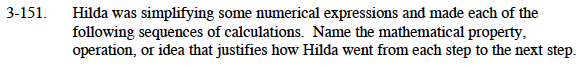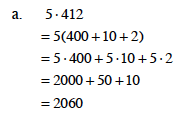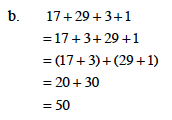### Home > MC1 > Chapter 3 > Lesson 3.4.5 > Problem3-151

3-151.
1. . Hilda was simplifying some numerical expressions and made each of the following sequences of calculations. Name the mathematical property, operation, or idea that justifies how Hilda went from each step to the next step. Homework Help ✎

1. 5 · 412
= 5(400 + 10 + 2)
= 5 · 400 + 5 · 10 + 5 · 2
= 2000 + 50 + 10
= 2060

2. 17 + 29 + 3 + 1
= 17 + 3 + 29 + 1
= (17 + 3) + (29 + 1)
= 20 + 30
= 50Decomposed 412
Distributive Property
Multiplied each term